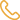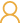877.235.4869Contact Us
Education

## How to find the right return on a rental property

By Nat Kunes January 9 2020 5 min read

Real estate investors sometimes have multiple reasons for investing in this asset class, but everyone who gets involved in real estate is ultimately interested in the returns that their investment properties provide. Afterall, all investment properties are ultimately investments and intended to provide a return.

Depending on how they purchase investment properties, there are a few different ways investors might calculate the return on investment (ROI) of their properties. Here are some tips for calculating the rate of return on a rental property according to three commonly used methods.

### The Simple Formula Helps Estimate Returns

The simple formula is the most basic ROI formula that real estate investors (and other investors) use to determine how well their holdings perform. To calculate a property’s ROI according to this formula, you simply divide the net returns by the cost of the investment. The exact formula is as follows:

• ROI = (Total Gains – Total Costs) / Total Costs x 100%

For example, assume you purchased and sold a single-family house. Also, figure your total expenses for the property were \$80,000 and it provided revenue of \$110,000. The costs would include your purchase price, any improvements, all maintenance, and any other expenses. The revenue would include all rents, the sale price, and any other form of income.

According to the simple formula, the total ROI of this property would be 37.5%. Here’s the equation: (\$110,000 – \$80,000) / \$80,000 x 100%.

The simple formula is a basic formula that can be useful if you want to know the actual ROI of specific holdings. It’s most helpful, however, when you want a basic estimation of ROI for a property. Carrying out this calculation is simple and you can also estimate the numbers. For a more precise calculation, though, one of the other two formulas below is often more useful.

### The Cap Rate Calculation is for Outright Ownership

A property’s cap rate provides a view of how much the property earns each year compared to what it costs to purchase. The rate is a ratio (expressed as a percentage) of net annual income to purchase price. The cap rate calculation formula is as follows:

• Cap rate = Annual Net Operating Income / Purchase Price x 100%

Of course, the annual net operating income is calculated by subtracting annual expenses from annual revenues.

To see how this might play out, assume the \$80,000 house from the previous example was rented for \$650 per month, or \$7,800 per year. Also, assume the house had an annual operating expenses of \$1,500, which would reduce the net operating income of the year to \$6,300.

With these numbers, the cap rate calculation would come out to 7.875%. The equation is (\$7,800 – \$1,500) / \$80,000 x 100%.

While most real estate investors are familiar with the cap rate calculation, those who recommend it among their main tips for calculating the rate of return on a rental property tend to purchase properties with cash. This formula is highly useful when a property is held outright, and it’s less insightful when a property is financed, because the formula accounts for the total price of a property — not just whatever an investor puts in.

### The Cash on Cash Calculation is for Financed Ownership

The cash on cash calculation is more useful when a rental property is financed with a bank or other institution (including an individual lender in a managed group setting). This formula better shows ROI based on what investors actually pay for a property. The cash on cash formula is:

• Cash on Cash Return = Annual Cash Flow / Total Cash Invested 100%

The example of the \$80,000 house once again shows how this calculation takes a loan into account. Assume that the house rents for \$650 per month and has annual expenses of \$1,500, but add on a mortgage of \$60,000 that has a monthly payment of \$375.

Based on these figures, the annual cash flow would be reduced to \$1,800 (\$7,800 annual rents – \$1,500 annual expenses – \$4,500 annual mortgage payments). Because only \$20,000 was put into the property, however, the cap rate is 9%. The equation is \$1,800 / \$20,000 x 100%.

### Use These Tips for Calculating the Rate of Return on a Rental Property

Are you considering a rental property or want to more fully see the rate of return on one you currently own? With the right numbers, calculating the rate of return on a rental property is easy, and can show you just how well your investment is performing. Choose the formula that is the most appropriate for your property, and you’ll be able to better understand your ROI.